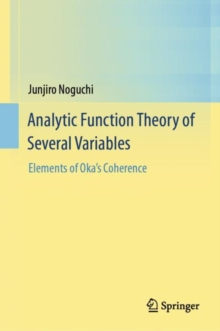# Analytic Function Theory of Several Variables : Elements of Oka's Coherence Hardback

#### Description

The purpose of this book is to present the classical analytic function theory of several variables as a standard subject in a course of mathematics after learning the elementary materials (sets, general topology, algebra, one complex variable).

This includes the essential parts of Grauert-Remmert's two volumes, GL227(236) (Theory of Stein spaces) and GL265 (Coherent analytic sheaves) with a lowering of the level for novice graduate students (here, Grauert's direct image theorem is limited to the case of finite maps).The core of the theory is "Oka's Coherence", found and proved by Kiyoshi Oka.

It is indispensable, not only in the study of complex analysis and complex geometry, but also in a large area of modern mathematics.

In this book, just after an introductory chapter on holomorphic functions (Chap. 1), we prove Oka's First Coherence Theorem for holomorphic functions in Chap. 2. This defines a unique character of the book compared with other books on this subject, in which the notion of coherence appears much later.The present book, consisting of nine chapters, gives complete treatments of the following items: Coherence of sheaves of holomorphic functions (Chap. 2); Oka-Cartan's Fundamental Theorem (Chap. 4); Coherence of ideal sheaves of complex analytic subsets (Chap. 6); Coherence of the normalization sheaves of complex spaces (Chap. 6); Grauert's Finiteness Theorem (Chaps. 7, 8); Oka's Theorem for Riemann domains (Chap. 8). The theories of sheaf cohomology and domains of holomorphy are also presented (Chaps. 3, 5). Chapter 6 deals with the theory of complex analytic subsets.

Chapter 8 is devoted to the applications of formerly obtained results, proving Cartan-Serre's Theorem and Kodaira's Embedding Theorem.

In Chap. 9, we discuss the historical development of "Coherence".It is difficult to find a book at this level that treats all of the above subjects in a completely self-contained manner.

In the present volume, a number of classical proofs are improved and simplified, so that the contents are easily accessible for beginning graduate students.

#### Information

• Format: Hardback
• Pages: 397 pages, 27 Illustrations, color; 1 Illustrations, black and white; XVIII, 397 p. 28 illus., 27 il
• Publisher: Springer Verlag, Singapore
• Publication Date:
• Category: Mathematical foundations
• ISBN: 9789811002892

£64.99

£49.88

Free delivery within the UK£85.50

£72.68

Available for